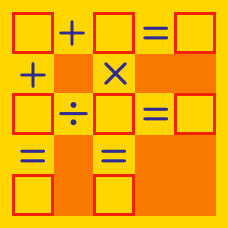Logic

Fill in the Blanks Warmup

Can we place a single digit in each $\square$, such that the following equation is true:

$\square \, \times \, \square = 11 ?$

What (possibly different) single digit should we place in $\square$ and $\bigcirc$ to make the following equation true:

$8 \square \div \bigcirc 8 = 3$

Give your answer as $( \square, \bigcirc )$.

This is an arithmetic puzzle, where $8 \square$ would represent the 2-digit number 89 if $\square = 9$. It does not represent the algebraic expression $8 \times \square$. The same logic applies for the 2-digit integer $\bigcirc 8$.

Does there exist (possibly different) digits which we can replace the $\square$ with, to make the following expression true:

$\square 2 \times 4 = \square 8 ?$

If in each of the squares, we must fill in a distinct digit, what is the minimum possible positive value of the difference?

$\begin{array} { l l l } & \square & \square \\ - & \square & \square \\ \hline \end{array}$

What identical digit can we place in $\square$, to make the following statement true:

$\Large 1 \square \times \square = \square 9 ?$

×